## tkinter -- Grid

实现机制是将 Widget 逻辑上分割成表格，在 指定的位置放置想要的 Widget 就可以了

第一个 Grid 例子

使用 grid 来布局组件

代码:

```import tkinter as tk
root = tk.Tk()
# 创建两个 Label
lb1 = tk.Label(root, text='hello')
lb2 = tk.Label(root, text='grid')
lb1.pack()
lb2.pack()
root.mainloop()```

结果: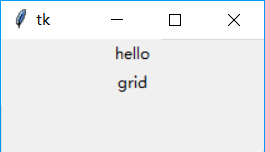grid 有两个最为重要的参数，用来指定将组件放置到什么位置，一个是 row,另一个是column。如果不指定 row,会将组件放置到第一个可用的行上，如果不指定 column，则使用第一列

使用 row 和 column 来指定位置

使用 grid 来布局组件

代码:

```import tkinter as tk
root = tk.Tk()
# 创建两个 Label
lb1 = tk.Label(root, text='hello')
lb2 = tk.Label(root, text='grid')

lb1.grid()
# 指定 lb2为第一行（使用索引0开始），第二列（使用索引0开始）
lb2.grid(row=0, column=1)
root.mainloop()```

结果: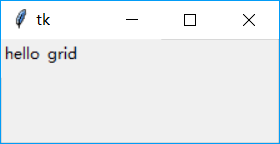grid 有两个最为重要的参数，用来指定将组件放置到什么位置，一个是 row,另一个是column。如果不指定 row,会将组件放置到第一个可用的行上，如果不指定 column，则使用第一列。注意这里使用 grid 时不需要创建，直接使用行列就可以

将两个或多个组件同一个位置

多个组件同时 grid 到同一个表格位置

代码:

```import tkinter as tk
root = tk.Tk()
# 创建两个 Label
lb1 = tk.Label(root, text='hello1')
lb2 = tk.Label(root, text='hello2')

# 将 lb1和 lb2均 grid 到(0,0)位置
lb1.grid(row=0, column=0)
lb2.grid(row=0, column=0)

def forgetLabel():
# grid_slaves 返回 grid 中(0,0)位置的所有组件
print(root.grid_slaves(0, 0))

# grid_salves 返回的第一个值为 lb2，最后 grid 的那一个
tk.Button(root, text='forget last', command=forgetLabel).grid(row=1)

root.mainloop()```

结果: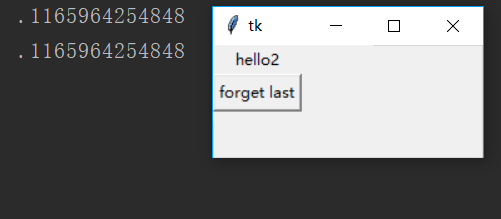改变列（行）的属性值

设置 column 的属性(columnconfigure)

代码:

```import tkinter as tk
root = tk.Tk()
# 创建两个 Label
lb1 = tk.Label(root, text='1', bg='red')
lb2 = tk.Label(root, text='2', bg='blue')

# 将 lb1和 lb2分别放置到第1行的1,2列位置上
lb1.grid(row=0, column=0)
lb2.grid(row=0, column=1)

# 指定列的最小宽度为100
root.columnconfigure(0, minsize=100)
root.mainloop()```

结果: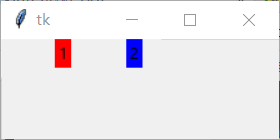1与2的距离变的远一些了。但如果这个位置没有组件存在的话这个值是不起作用的.设置列或行(rowconfigure)的属性时使用父容器的方法,不是自己调用

组件使用多列（多行）

使用多行（多列)

代码:

```import tkinter as tk
root = tk.Tk()
# 创建5个 Label，分别以背景色区别
lb1 = tk.Label(root,text='a', bg='red')
lb2 = tk.Label(root,text='b', bg='blue')
lb3 = tk.Label(root,text='c', bg='red')
lb4 = tk.Label(root,text='d', bg='blue')
lb5 = tk.Label(root,text='e', bg='red')

# 以下为布局参数设置
lb1.grid(row=0, column=0, columnspan=2)
lb2.grid(row=1, column=0)
lb3.grid(row=1, column=1)
lb4.grid(row=2)
lb5.grid(row=0, column=2)

root.mainloop()```

结果:A 与 B、D 的区别，它左边已改变，由于使用了两个表格；C 与 E 的区别：C 的右边与 E 的左边对齐，也就是说 E 被放置到第2列的下一个位置了，原因由于 A 已使用了第2列

设置表格中组件的对齐属性

使用 sticky 设置对齐方式

代码:

```import tkinter as tk
root = tk.Tk()
# 创建两个 Label
tk.Label(root, text='hello sticky').grid()
tk.Label(root, text='Tkinter').grid()

# 创建两个 Label，并指定 sticky 属性
tk.Label(root, text='hello sticky').grid(sticky='w')
tk.Label(root, text='Tkinter').grid(sticky='w')

root.mainloop()```

结果: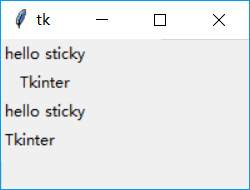默认属性下，组件的对齐方式为居中，设置 sticky 属性可以控制对齐方式，可用的值（N,S,E,W)及其组合值